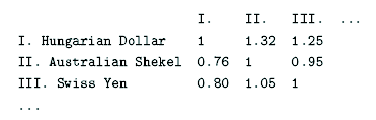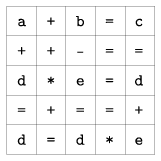Mathematical and Physical Journal
for High Schools
Issued by the MATFUND Foundation
 Already signed up? New to KöMaL?

# KöMaL Problems in Informatics, December 2007

Show/hide problems of signs:## Problems with sign 'I'

Deadline expired on January 15, 2008.

I. 172. There is a winter sale'' at a shop, so one gets a voucher of value 100 HUF if one purchases goods for more than 3000 HUF. The voucher can be redeemed at a next purchase. We plan to buy some items in that shop and want to exploit those vouchers optimally: we may visit the shop more than once and regroup the items as necessary in order to minimize the total amount we pay, that is, to get the maximal number of vouchers.

Prepare your program to group items (at most 100) to be bought, such that we get the maximal number of vouchers (that is, the total amount we pay is minimal). Each line of the input text file contains the identifier of each item (a 6-digit number) and its price (between 10 HUF and 50 000 HUF) separated by a space. The first command line argument of your program is the name of the input file, while the second argument is the name of the output file. The output file should only contain the amounts to pay at different purchases, each occasion in a separate line, finally, the total amount in the last line.

The source code of your program (i172.pas, i172.cpp, ...) together with a short documentation (i172.txt, i172.pdf, ...) should be submitted, also containing a short description of your solution and the name of the developer environment to use.

(10 pont)

solution (in Hungarian), statistics

I. 173. There were 14 questions in a pool, each with a possible answer of 1'', 2'' or X''. We would like to rank the competitors who played this game. First we assign a certain number to each question: a question is worth m points if there were m people not answering correctly that question. A competitor answering correctly a question gets the number of points of that question. The winner(s) is/are who get(s) the maximal number of points altogether.

Prepare a spreadsheet application to evaluate the pool. Beginning with the first row, each line contains the name of the contestant, then his or her 14 answers in the first 15 columns of the Answers'' sheet. There are at most 500 rows. If someone gave no answer to a question, that cell would remain empty. For computations and for the output, you can use the Computations'' sheet: cell A1 should contain the maximal number of points achieved, while cell B1 the name(s) of the winner(s).

You should submit the spreadsheet (i173.xls, i173.ods, ...) and a short documentation (i173.txt, i173.pdf, ...) containing a description of your solution, further, the name and version number of your spreadsheet application.

(10 pont)

solution (in Hungarian), statistics

I. 174. Money Eater Ltd. deals with currency exchange. The boss wants all employees to have access to all exchange rates, so he uses a web page with the following structure:

...
<table>
<tr><td>Hungarian Dollar</td>
<td>100</td></tr>
<tr><td>Australian Shekel</td>
<td>76</td></tr>
<tr><td>Swiss Yen</td>
<td>80
...
</table>
...

The first row of the table is the base currency, while other exchange rates are quoted in terms of this currency. In the example, 100 Hungarian Dollar correspond to 76 Australian Shekel or 80 Swiss Yen.

Write a program that converts exchange rates given in an HTML file (the input of your program) to a TXT file (the output of your program), so, for example, a command line could be i174 in.html out.txt.

The output of the above example would be the following TXT file:The source code of your program (i174.pas, i174.cpp, ...) together with a short documentation (i174.txt, i174.pdf, ...) should be submitted, also containing a short description of your code and the name of the developer environment to use.

(10 pont)

solution (in Hungarian), statistics## Problems with sign 'S'

Deadline expired on May 15, 2008.

S. 31. Someone made up a 5×5 table containing equations. Each cell of the table contains either a basic arithmetic operation (any of the 4 operations), a variable (a single letter) or the equality sign.

See the example:When reading rows (from left to right), columns and the two diagonals (downwards) of the table, we get a system of 8 equations. During the solution of this system, one can always find an equation from which a suitable unknown can be expressed (independently of the other equations), or a suitable simple relation between two unknowns can be derived.

In the example above, one sees from the last line that d=0 or e=1. The first column implies a=0, so b=c due to the first row. Proceeding in a similar way, the solution is a=d=0 with b=c=e=arbitrary; or a=0, e=1 and b=c=1+d with d arbitrary.

Write your program to solve an arbitrary (syntactically correct) table. The first input parameter of your program is the name of the text file containing the table. The input file consists of 5 rows. Each row contains the successive entries of a row in the table (that is, mathematical symbols or unknowns) separated by a space. The output of your program on the standard output should consist of a list of values of the variables in alphabetical order (e.g. a=3.25'' or the value of a is arbitrary''). If the system can not be solved, a warning should be issued.

The source code of your program (s31.pas, s31.cpp, ...) together with a short documentation (s31.txt, s31.pdf, ...) should be submitted, also containing a short description of your code and the name of the developer environment to use.

Proposed by András Tálas, 12th grade student, Budapest

(10 pont)

statistics

\$Var(Body)